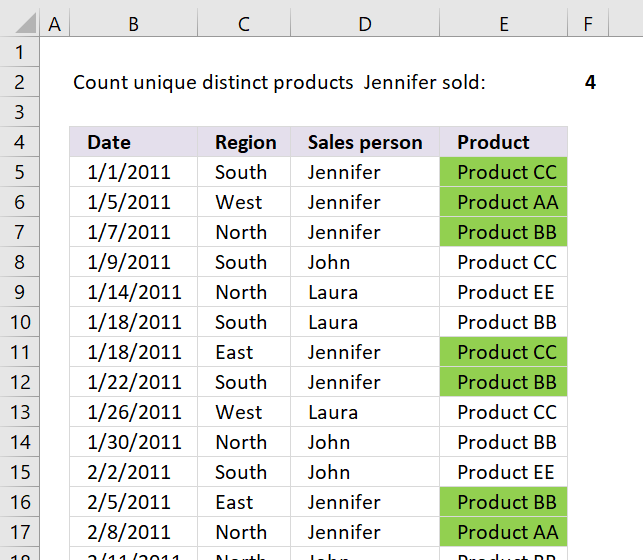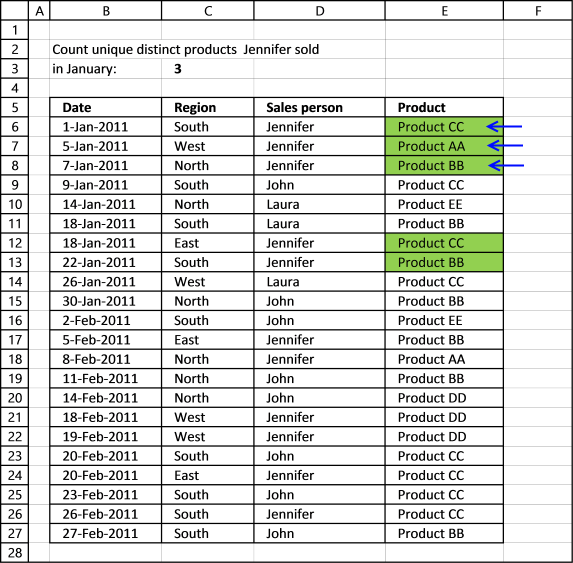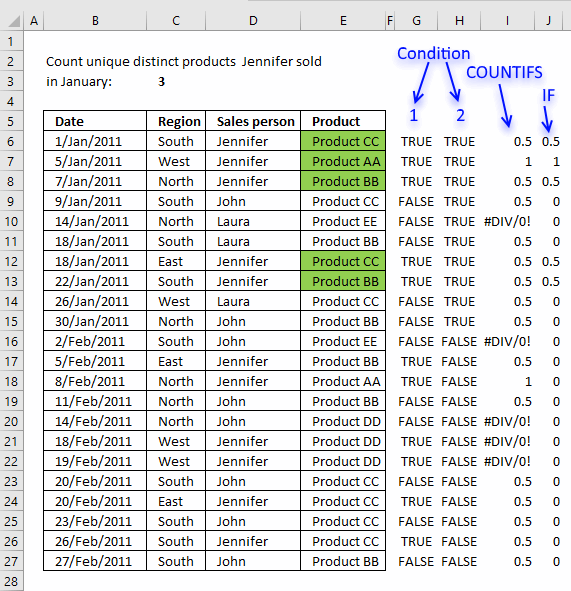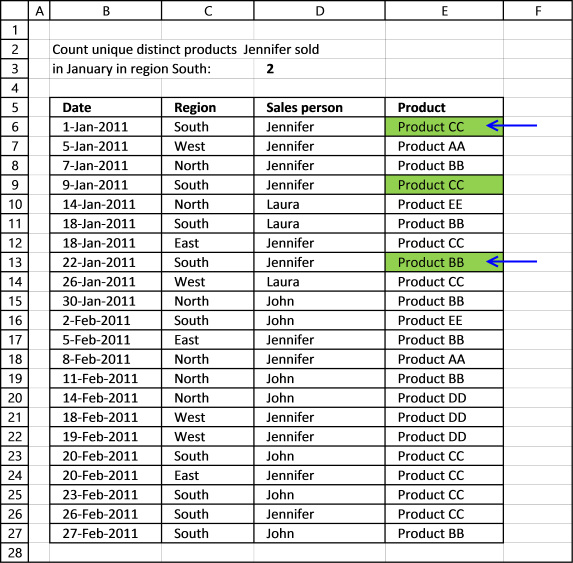Author: Oscar Cronquist Article last updated on January 24, 2019This post demonstrates how to build an array formula that counts unique distinct values based on criteria.

Unique distinct values are all values except that duplicates are merged into one value, in other words, duplicates are removed.

I highly recommend you use a pivot table if you own Excel 2013 or a later version.

A pivot table is easier to work with and much faster if you have lots of data:
Count unique distinct values [Pivot Table]

An array formula is great for an interactive dashboard or dynamic data meaning data changes often, like once a week or perhaps once a month.

If you use an Excel defined Table or a dynamic named range you can quickly change the data range without editing the cell references in the array formula.

### How to count unique distinct items based on a condition and a date condition?How many unique distinct products did Jennifer sell in January?

Array formula in C3:

=SUM(IF(("Jennifer"=\$D\$6:\$D\$27)*(\$B\$6:\$B\$27<=DATE(2011, 1, 31)), 1/COUNTIFS(\$D\$6:\$D\$27, "Jennifer", \$E\$6:\$E\$27, \$E\$6:\$E\$27, \$B\$6:\$B\$27, "<="&DATE(2011, 1, 31))), 0))

#### Watch this video where I explain how the above formula works

This calculation is also possible using a pivot table, you simply add more criteria: Count unique distinct values [Pivot Table]

### Explaining formula in cell C3

#### Step 1 - Find values meeting first condition

The equal sign compares the condition (Jennifer) to all cell values in \$D\$6:\$D\$27 and returns an array containing TRUE or FALSE (boolean values).

("Jennifer"=\$D\$6:\$D\$27)

becomes

"Jennifer"={"Jennifer"; "Jennifer"; "Jennifer"; "John"; "Laura"; "Laura"; "Jennifer"; "Jennifer"; "Laura"; "John"; "John"; "Jennifer"; "Jennifer"; "John"; "John"; "Jennifer"; "Jennifer"; "John"; "Jennifer"; "John"; "Jennifer"; "John"}

and returns

{TRUE; TRUE; TRUE; FALSE; FALSE; FALSE; TRUE; TRUE; FALSE; FALSE; FALSE; TRUE; TRUE; FALSE; FALSE; TRUE; TRUE; FALSE; TRUE; FALSE; TRUE; FALSE}

#### Step 2 - Find values meeting the second condition

The less than < and equal sign together lets you compare Date 1/31/2011 with dates in \$B\$6:\$B\$27, it returns TRUE if the date is earlier than or equal to 1/31/2011.

(\$B\$6:\$B\$27<=DATE(2011, 1, 31))

becomes

({40544; 40548; 40550; 40552; 40557; 40561; 40561; 40565; 40569; 40573; 40576; 40579; 40582; 40585; 40588; 40592; 40593; 40594; 40594; 40597; 40600; 40601}<=DATE(2011, 1, 31))

becomes

({40544; 40548; 40550; 40552; 40557; 40561; 40561; 40565; 40569; 40573; 40576; 40579; 40582; 40585; 40588; 40592; 40593; 40594; 40594; 40597; 40600; 40601}<=40574)

and returns

{TRUE; TRUE; TRUE; TRUE; TRUE; TRUE; TRUE; TRUE; TRUE; TRUE; FALSE; FALSE; FALSE; FALSE; FALSE; FALSE; FALSE; FALSE; FALSE; FALSE; FALSE; FALSE}

#### Step 3 - Calculate the number of records that contain condition 1 and 2 and any products

The COUNTIFS function calculates the number of cells across multiple ranges that equals all given conditions. There is a criteria range and a condition forming a pair.

 Pair Criteria range Criteria 1 \$D\$6:\$D\$27 "Jennifer" 2 \$E\$6:\$E\$27 \$E\$6:\$E\$27 3 \$B\$6:\$B\$27 "<="&DATE(2011, 1, 31)

1/COUNTIFS(\$D\$6:\$D\$27, "Jennifer", \$E\$6:\$E\$27, \$E\$6:\$E\$27, \$B\$6:\$B\$27, "<="&DATE(2011, 1, 31)))

becomes

1/{2; 1; 2; 2; 0; 2; 2; 2; 2; 2; 0; 2; 1; 2; 0; 0; 0; 2; 2; 2; 2; 2}

and returns

{0.5; 1; 0.5; 0.5; #DIV/0!; 0.5; 0.5; 0.5; 0.5; 0.5; #DIV/0!; 0.5; 1; 0.5; #DIV/0!; #DIV/0!; #DIV/0!; 0.5; 0.5; 0.5; 0.5; 0.5}

#### Step 4 - If condition 1 and 2 are TRUE then return numbers from step 3

The IF function has three arguments, the first one must be a logical expression. If the expression evaluates to TRUE then one thing happens (argument 2) and if FALSE another thing happens (argument 3).

IF(("Jennifer"=\$D\$6:\$D\$27)*(\$B\$6:\$B\$27<=DATE(2011, 1, 31)), 1/COUNTIFS(\$D\$6:\$D\$27, "Jennifer", \$E\$6:\$E\$27, \$E\$6:\$E\$27, \$B\$6:\$B\$27, "<="&DATE(2011, 1, 31))), 0)

becomes

IF({TRUE; TRUE; TRUE; FALSE; FALSE; FALSE; TRUE; TRUE; FALSE; FALSE; FALSE; TRUE; TRUE; FALSE; FALSE; TRUE; TRUE; FALSE; TRUE; FALSE; TRUE; FALSE}*{TRUE; TRUE; TRUE; TRUE; TRUE; TRUE; TRUE; TRUE; TRUE; TRUE; FALSE; FALSE; FALSE; FALSE; FALSE; FALSE; FALSE; FALSE; FALSE; FALSE; FALSE; FALSE}, 1/COUNTIFS(\$D\$6:\$D\$27, "Jennifer", \$E\$6:\$E\$27, \$E\$6:\$E\$27, \$B\$6:\$B\$27, "<="&DATE(2011, 1, 31))), 0)

becomes

IF({1;1;1;0;0;0;1;1;0;0;0;0;0;0;0;0;0;0;0;0;0;0}, 1/COUNTIFS(\$D\$6:\$D\$27, "Jennifer", \$E\$6:\$E\$27, \$E\$6:\$E\$27, \$B\$6:\$B\$27, "<="&DATE(2011, 1, 31))), 0)

You get the numerical equivalents if you add or multiply arrays, the numerical equivalent of TRUE is 1 and FALSE is 0 (zero),

IF({1;1;1;0;0;0;1;1;0;0;0;0;0;0;0;0;0;0;0;0;0;0}, 1/COUNTIFS(\$D\$6:\$D\$27, "Jennifer", \$E\$6:\$E\$27, \$E\$6:\$E\$27, \$B\$6:\$B\$27, "<="&DATE(2011, 1, 31))), 0)

becomes

IF({1;1;1;0;0;0;1;1;0;0;0;0;0;0;0;0;0;0;0;0;0;0}, {0.5; 1; 0.5; 0.5; #DIV/0!; 0.5; 0.5; 0.5; 0.5; 0.5; #DIV/0!; 0.5; 1; 0.5; #DIV/0!; #DIV/0!; #DIV/0!; 0.5; 0.5; 0.5; 0.5; 0.5}, 0)

and returns

{0.5; 1; 0.5; 0; 0; 0; 0.5; 0.5; 0; 0; 0; 0; 0; 0; 0; 0; 0; 0; 0; 0; 0; 0}The image above shows the results of each step.

• Step 1 - Column G
• Step 2 - Column H
• Step 3 - Column I
• Step 4 - Column J

#### Step 5 - Sum numbers in array

SUM(IF("Jennifer"=\$D\$5:\$D\$26, 1/(COUNTIFS(\$D\$5:\$D\$26, "Jennifer", \$E\$5:\$E\$26, \$E\$5:\$E\$26)), 0))

becomes

SUM({0.5; 1; 0.5; 0; 0; 0; 0.5; 0.5; 0; 0; 0; 0; 0; 0; 0; 0; 0; 0; 0; 0; 0; 0})

and returns 3 in cell C3. There are three unique distinct products based on two conditions.

Recommended article:

Count unique distinct records

The image above shows a table with 3 columns containing random data. It is quite complicated trying to manually count […]

### Example 2,

How many unique distinct products did Jennifer sell in January and in region South?Array formula in D3:

=SUM(IF(("Jennifer"=\$D\$6:\$D\$27)*(\$B\$6:\$B\$27<=DATE(2011, 1, 31))*("South"=\$C\$6:\$C\$27), 1/COUNTIFS(\$D\$6:\$D\$27, "Jennifer", \$E\$6:\$E\$27, \$E\$6:\$E\$27, \$B\$6:\$B\$27, "<="&DATE(2011, 1, 31), \$C\$6:\$C\$27, "South")), 0)

Recommended article:

Count unique distinct values based on a condition

The following article demonstrates how to construct a formula that counts unique distinct values based on a condition. The image […]

### Example 3,

How many unique distinct products was sold in the south or in January?

Array formula:

=SUM(--(FREQUENCY(IF(((\$B\$10:\$B\$31<=DATE(2011, 1, 31))*(\$B\$10:\$B\$31>=DATE(2011, 1, 1)))+("South"=\$C\$10:\$C\$31), COUNTIF(\$E\$10:\$E\$31, "<"&\$E\$10:\$E\$31), ""), COUNTIF(\$E\$10:\$E\$31, "<"&\$E\$10:\$E\$31))>0))

### Download Excel fileCount-unique-distinct-values-meeting-criteria.xlsx

Recommended article:

Count dates inside a date range

How do I automatically count dates in a specific date range? Array formula in cell D3: =SUM(IF((\$A\$2:\$A\$10<\$D\$2)*(\$A\$2:\$A\$10>\$D\$1), 1, 0)) + […]

Count unique distinct values in a filtered Excel defined Table

This article demonstrates a formula that counts unique distinct values filtered from an Excel defined Table. Debra Dalgleish described in […]